A versatile cross-platform mind mapping tool.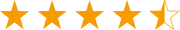Get from App StoreA versatile cross-platform mind mapping tool.Get from App Store
edraw-max-horizontal
Product Tour
Templates
Guide
What's New
EdrawMax Online
TRY IT FREE

# Drawing Basics

> Drawing Basics
Posted by James Freeman | 12/23/2019
Vector graphics are made up of lines and curves defined by mathematical objects called vectors, which describe an image according to its geometric characteristics.

Vector graphics (sometimes called vector shapes or vector objects) are made up of lines and curves defined by mathematical objects called vectors, which describe an image according to its geometric characteristics.

Vector graphics are resolution-independent - they maintain crisp edges when resized, printed to a PostScript printer, saved in a PDF file, or imported into a vector-based graphics application. As a result, vector graphics are the best choice for artwork, such as logos, which will be used at various sizes and in various output media.

You can use drawing tools to create vector objects and save them as library elements.

As you draw, you create a line called a path. A path is made up of one or more straight or curved segments. The beginning and end of each segment are marked by anchor points which work like pins holding a wire in place. A path can be closed (for example, a circle), or open, with distinct endpoints (for example, a wavy line).

You change the shape of a path by dragging its anchor points, the direction points at the end of direction lines that appear at anchor points, or the path segment itself.

#### Components of a Path

A. Unselected anchor point  B. Selected anchor point  C. Direction point  D. Direction line  E. Straight segment  F. Curved segment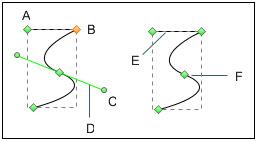Paths can have two kinds of anchor points: corner points and smooth points. At a corner point, a path abruptly changes direction. At a smooth point, path segments are connected as a continuous curve. You can draw a path using any combination of corner and smooth points. If you draw the wrong kind of point, you can always change it.

#### Points on a Path

A. Four corner points  B. Four smooth points  C. Combination of corner and smooth points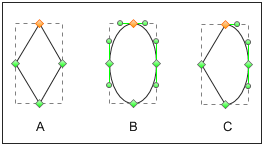A corner point can connect any two straight or curved segments, while a smooth point always connects two curved segments.

A corner point can connect both straight segments and curved segments.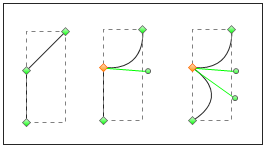### About Direction Lines and Direction Points

When you select an anchor point that connects curved segments (or select the segment itself), the anchor points of the connecting segments display direction handles, which consist of direction lines that end in direction point. The angle and length of the direction lines determine the shape and size of the curved segments. Moving the direction points reshapes the curves. Direction lines don't appear in the final output.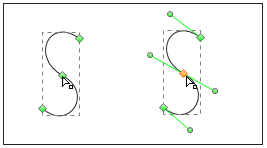After selecting an anchor point (left), direction lines appear on any curved segments connected by the anchor point (right).

A smooth point always has two direction lines, which move together as a single, straight unit. When you move a direction line on a smooth point, the curved segments on both sides of the point are adjusted simultaneously, maintaining a continuous curve at that anchor point.

In comparison, a corner point can have two, one, or no direction lines, depending on whether it joins two, one, or no curved segments, respectively. Corner point direction lines maintain the corner by using different angles. When you move a direction line on a corner point, only the curve on the same side of the point as that direction line is adjusted.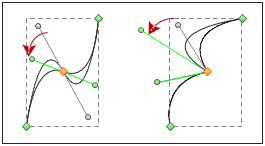Adjusting direction lines on a smooth point (left) and a corner point (right)

Direction lines are always tangent to (perpendicular to the radius of) the curve at the anchor points. The angle of each direction line determines the slope of the curve, and the length of each direction line determines the height or depth of the curve.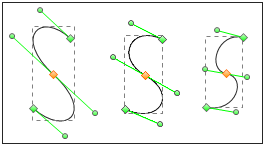Moving and resizing direction lines change the slope of curves.

Manage Diagrams